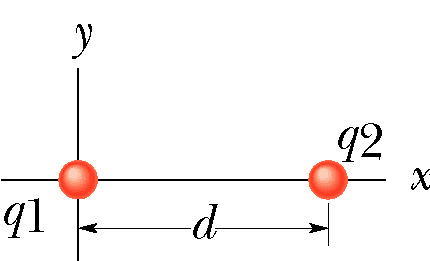# Find Net Electric Field Between Two Point Charges

## Homework Statement

In the figure, two point charges q1 = 3.40×10-5 C and q2 = 1.70×10-4 C are separated by a distance d = 0.10 m. Compute their net electric field E (x) as a function of x for the following positive and negative values of x, taking E to be positive when the vector E points to the right and negative when E points to the left. Plot your values in your notebook.
What is E(-0.100)?## Homework Equations

E(X)=k[(q1/x^2)-(q2/(d+x)^2)]

## The Attempt at a Solution

I used the above equation, but the answer was wrong. When E(-0.100), does that mean the x in the equation is also negative? I get the feeling I've messed up some of the signs.

BvU
Homework Helper
There are two things wrong with you relevant equation.
1. If you want the magnitude of the field due to a charge, you need the distance to that charge. That is correct for q1 in your equation, but not for q2
2. The minus sign in your equation is only valid in 0 < x < d because there the field from q1 points to the right (positive x direction) and from q2 to the left.

When it says E(-0.100), does that mean the x value of -0.100, as in a point on the x axis to the left of q1? (-0.100, 0)

For your first point, are you saying that it should be (d-x)^2?
E(X)=k[(q1/x^2)+(q2/(d-x)^2)]

BvU
Homework Helper
When it says E(-0.100), does that mean the x value of -0.100, as in a point on the x axis to the left of q1? (-0.100, 0) -- Yes

For your first point, are you saying that it should be (d-x)^2? -- Yes. Better: (x-d)2

Second point, that the two fractions should instead be added? --- Yes/No:

To the right of a charge q/x2 points to the right
To the left of a charge q/x2 points to the left​

To get it in one expression, we often use this notation : ##\vec E(\vec r) = k\; q\; {\displaystyle \vec r\over |\vec r|^3}##

q itself also has a sign. In this exercise both q1 and q2 are positive. So

0<x<d q1 to the right, q2 to the left (as in your original relevant equation E(X)=k[(q1/x2) - (q2/(d-x)2)] )
d<x both point to the right => add (so indeed E(X)=k[(q1/x2) + (q2/(d-x)2)] )​

Make a drawing !

•rlc
E(x<0)=-k[(q1/x^2) + (q2/(d-x)^2)]
E(0<x<d)=k[(q1/x^2) - (q2/(d-x)^2)]
E(d<x)=k[(q1/x^2) + (q2/(d-x)^2)]

These equations all worked! Thank you so much for explaining it all to me, I think I actually understand now why you would subtract vs. add!